# Multiplication Using the Compensation Strategy

Multiplication Using the Compensation Strategy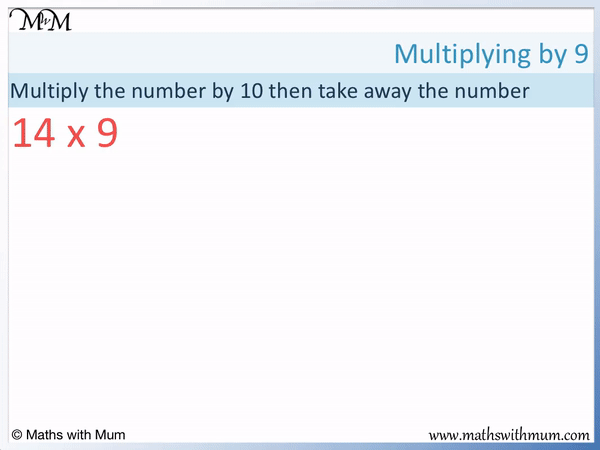• The compensation strategy for multiplication involves multiplying by a multiple of 10 and then subtracting lots of a number afterwards.
• For example, to multiply a number by 9, multiply it by 10 and then subtract 1 lot of that number.
• To work out 14 × 9, work out 14 × 10 and then subtract 1 lot of 14..
• 14 × 10 = 140. We can simply place a zero digit on the end to multiply a whole number by 10.
• We then subtract 1 lot of 14 because we want 9 lots of 14 not 10 lots.
• 140 – 14 = 126 and so, 14 × 9 = 126.
• We can use the compensation strategy to find other multiples by subtracting two or three lots of a number.
• To find 14 × 8, we would multiply 14 by 10 and subtract 2 lots of 14 because 8 is 2 less than 10.

Multiply a number by a multiple of 10 and then subtract as many lots of the number as you need to compensate.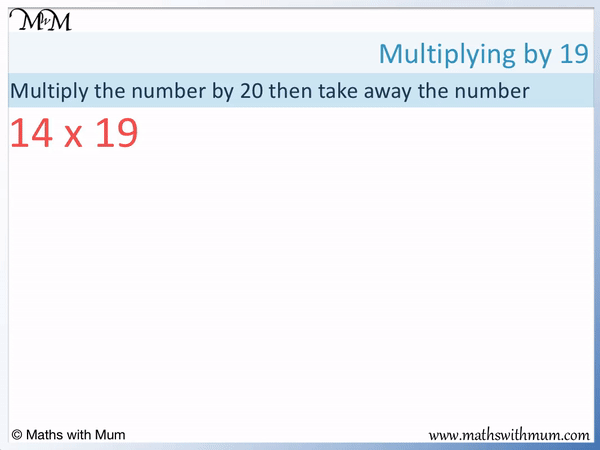• We want to multiply 14 × 19 using the compensation strategy.
• The next multiple of 10 after 19 is 20.
• We multiply 14 by 20 and then subtract 1 lot of 14 to find 14 × 19.
• We can find 14 × 20 by multiplying 14 by 2 and then by 10.
• 14 × 20 = 280. We need to subtract 1 lot of 14 to find 14 × 19
• 280 – 14 = 266 and so 14 × 19 = 266
• We subtracted 1 lot of the number away from 20 lots of the number because 20 – 1 = 19.Supporting Lessons#### Multiplication Compensation Strategy: Interactive Questions

Multiplication Compensation Strategy: Interactive Questions

# Multiplication Using the Compensation Strategy

## What is the Compensation Strategy for Multiplication?

The multiplication by compensation strategy is taught as a method for multiplying by numbers that are close to a multiple of 10, such as multiplying by 9. The method involves multiplying by a larger number that is a multiple of 10 and then subtracting amounts afterwards as necessary. The idea of subtracting an amount afterwards gives this method the name of compensation.

For example, here is 27 × 9. This is not a quick or easy calculation to do mentally or using long multiplication. Instead we will use the compensation method.

Instead of multiplying 27 by 9, we can multiply it by 10. This is chosen because multiplying a whole number by 10 is easy. We simply write a 0 digit on the end.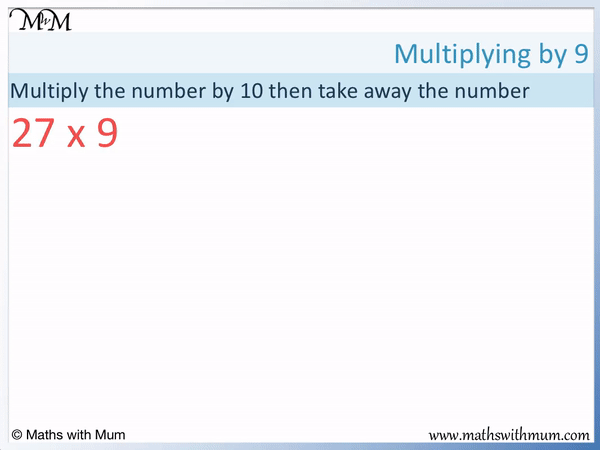27 × 10 = 270

We have found 10 lots of 27 although we only wanted 9 lots of 27.

9 is one less than 10 and so, we need to remove one lot of 27 from the 10 lots of 27 that we have found.

We simply subtract 1 lot of 27 from 270.

270 – 27 = 243 and so, 9 lots of 27 = 243 and 27 × 9 = 243.

This method was chosen because multiplying by 10 is easier than multiplying by 9. Subtraction is often an easier calculation to perform than a multiplication. The compensation method fof multiplying numbers is often taught as an extra strategy to speed up mental multiplication or to act as an alternative to calculating multiplications by hand.

The compensation method is used to multiply by a number that is close to a multiple of 10. The method can be used to multiply by 9, 8 or 7 as they are close to 10. It could also be used to multiply by 19, 18 or 17, which are close to 20 or to multiply by larger numbers such as 49, which is close to 50.

## How to Multiply Numbers Using the Compensation Strategy

To multiply a number using the compensation strategy, follow these steps:

1. Multiply the first number by the next multiple of 10 that comes after the second number.
2. Find the difference between the second number and the next multiple of 10 after it.
3. Subtract the first number from the answer to step 1 as many times as the difference found in step 2.

For example, here is 88 × 9.

The next multiple of 10 that comes after 9 is 10. We will first multiply 88 by 10.

88 × 10 = 880.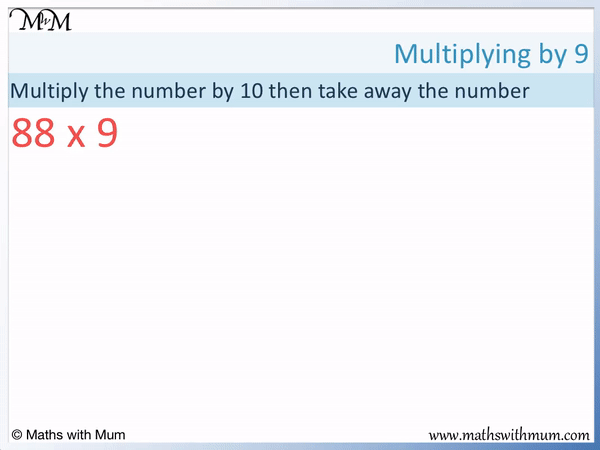The next step is to find the difference between the second number and the next multiple of 10 after it. The difference between 9 and 10 is 1.

We will subtract 88 once from 880. This is because 880 is 10 lots of 88 and we only need 9 lots of 88. We need to remove 1 lot of 88 from 880.

880 – 88 = 792 and therefore, 88 × 9 = 792

Here is another example of multiplying by compensation.

We have 22 × 19.

The first step is to find the next multiple of 10 that comes after 19. This is 20.

We multiply 22 by 20 instead of 19. To multiply by 20, multiply by 2 and then by 10.

22 × 2 = 44 and 44 × 10 = 440. Therefore 22 × 20 = 440.The next step is to find the difference between 19 and 20, which is 1. We need to subtract 1 lot of 22 from 440 because we want 19 lots of 22 not 20 lots.

440 – 22 = 418 and so 22 × 19 = 418.

## How to Multiply a Number by 9

To multiply a number by 9, multiply the number by 10 and subtract the number from the result.

For example, here is 145 × 9.

We multiply 145 by 10 by simply writing a 0 digit on the end. 145 × 10 = 1450.The next step is to subtract the number of 145 from this result.

1450 – 145 = `1305 and so 145 × 9 = 1305.

## How to Multiply a Number by 19

To multiply a number by 19, multiply the number by 20 and subtract the number from the result.

For example, here is 36 × 19.

We multiply 36 by 20 and then subtract 36.

We can multiply by 20 by multiplying by 2 and then by 10.

36 × 2 = 72 and then 72 × 10 = 720. 36 × 20 = 720.We now subtract 36 from this result.

720 – 36 = 684 and so, 36 × 19 = 684.Now try our lesson on Grid Method Multiplication where we learn how to use the grid method to multiply larger numbers.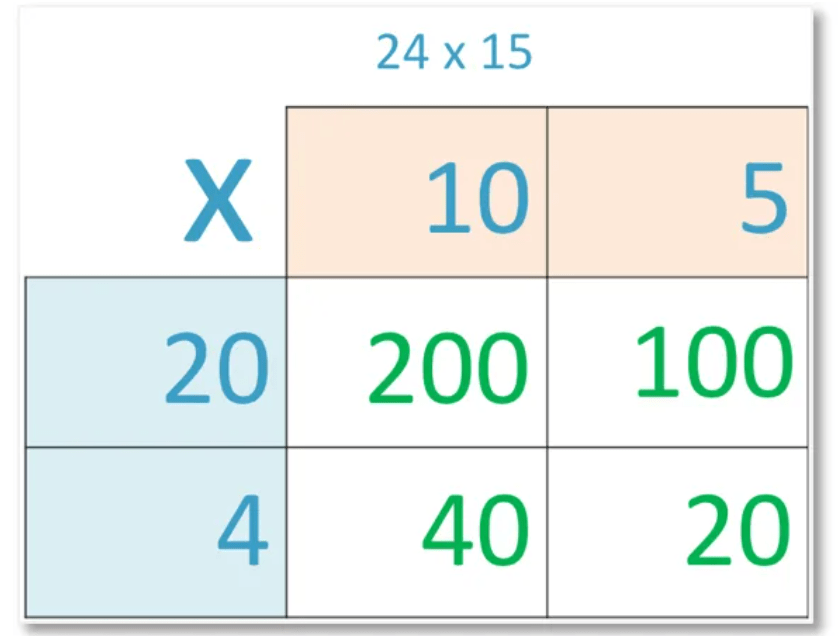error: Content is protected !!